## in a young’s double-slit experiment the center of a bright fringe occurs wherever waves from the slits differ in the distance they travel by

Question

in a young’s double-slit experiment the center of a bright fringe occurs wherever waves from the slits differ in the distance they travel by a multiple of

in progress 0
5 months 2021-08-02T21:14:50+00:00 1 Answers 92 views 0

The path difference will be zero.

Explanation:

Given that,

In a young’s double-slit experiment the center of a bright fringe occurs wherever waves from the slits differ in the distance.

Suppose, we find the multiple of wavelength

In young’s double slit experiment,

We need to calculate the multiple of wavelength

Using formula of path difference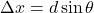….(I)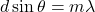Where, m = fringe number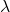= wavelength

For center of a bright fringe ,

m = 0

Put the value into the formula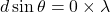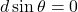Put the value in equation (I)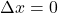Hence, The path difference will be zero.